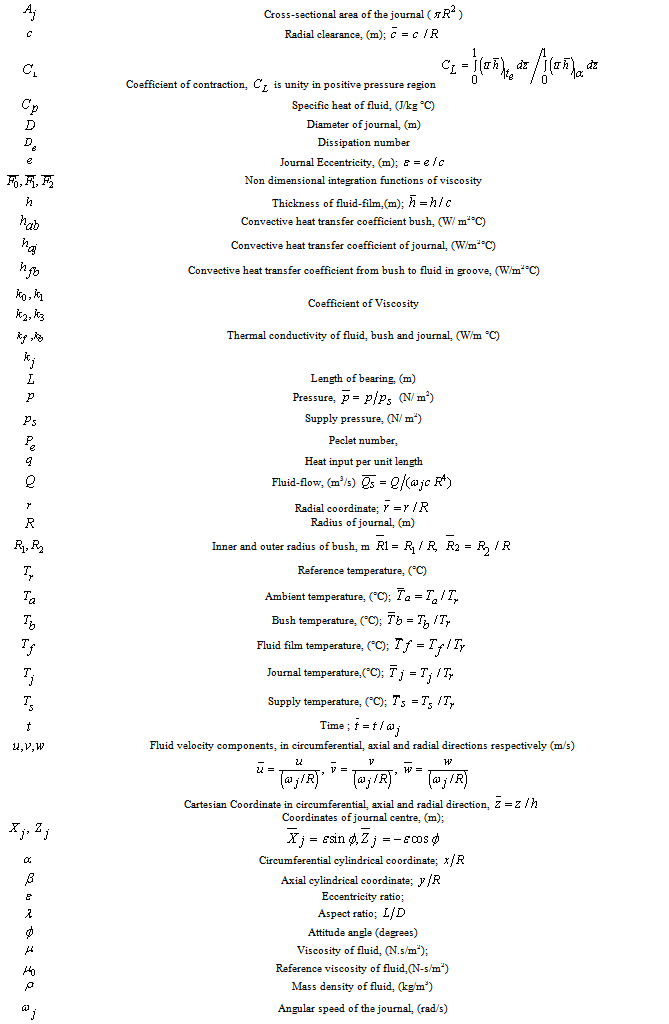﻿ The Influence of Modified Viscosity-Temperature Equation on Thermohydrodynamic Analysis of Plain Journal Bearing

### The Influence of Modified Viscosity-Temperature Equation on Thermohydrodynamic Analysis of Plain Jou...

K. R. Kadam, Dr. S. S. Banwait, Dr. S. C. LaroiyaOPEN ACCESSPEER-REVIEWED

## The Influence of Modified Viscosity-Temperature Equation on Thermohydrodynamic Analysis of Plain Journal Bearing

K. R. Kadam1,, Dr. S. S. Banwait2, Dr. S. C. Laroiya3

1Research Scholar, Mechanical Engineering Department, National Institute of Technical Teachers’ Training & Research Sector 26, Chandigarh, 160019, India

2Professor & Head of Mechanical Engineering Department, National Institute of Technical Teachers’ Training & Research, Sector 26, Chandigarh,160019, India

3Ex-Director, National Institute of Technical Teachers’ Training & Research, Sector 26, Chandigarh, 160019, India

### Abstract

In this paper a thermohydrodynamic analysis has been done to investigate the influence of modified viscosity-temperature equation on plain journal bearing. This analysis is based on the numerical solution of three-dimensional energy equation coupled with Reynolds equation and the heat conduction equation in the journal bearing. Reynolds equation for the pressure distribution and the energy equation for the temperature distribution were used for this analysis. Finite element method was used to solve the Reynolds equation. By solving the generalized Reynolds equation, hydrodynamic pressure was found out. Finite difference method was used for predicting temperature distribution in journal bearing. The Fourier heat conduction equation in the non-dimensional cylindrical coordinate has been adopted for finding the temperature distribution in the bush. The temperature distribution along axial direction of the journal has been found out using a steady-state unidirectional heat conduction equation.

### At a glance: Figures

12
Prev Next

• Kadam, K. R., Dr. S. S. Banwait, and Dr. S. C. Laroiya. "The Influence of Modified Viscosity-Temperature Equation on Thermohydrodynamic Analysis of Plain Journal Bearing." American Journal of Mechanical Engineering 2.6 (2014): 169-177.
• Kadam, K. R. , Banwait, D. S. S. , & Laroiya, D. S. C. (2014). The Influence of Modified Viscosity-Temperature Equation on Thermohydrodynamic Analysis of Plain Journal Bearing. American Journal of Mechanical Engineering, 2(6), 169-177.
• Kadam, K. R., Dr. S. S. Banwait, and Dr. S. C. Laroiya. "The Influence of Modified Viscosity-Temperature Equation on Thermohydrodynamic Analysis of Plain Journal Bearing." American Journal of Mechanical Engineering 2, no. 6 (2014): 169-177.

 Import into BibTeX Import into EndNote Import into RefMan Import into RefWorks

### 1. Introduction

Journal bearings are used in rotating heavy machinery. To obtain high efficiency and reliability in combustion engines and turbines journal bearings were used. A journal bearing consists of a shaft rotating within a bush. The hydrodynamic fluid film supports the load, which was generated between the shaft and the bush. Friction and wear of mating parts must be kept low. Most of the high speed rotating machinery fails due to failure of bearings. Due to the heavy load and high speed, the temperature increases in the bearing. An accurate thermohydrodynamic analysis is required to find the thermal response of the lubricating fluid and bush. Therefore, a need has been felt to carry out further investigation on the thermal effects in journal bearings.

Allan T.  utilized the variation calculus approach and shows how the appropriate matrix equations were derived. Finite element method used for solving the Reynolds equation. By considering thermal effects Dowson D. and Ashton J. N.  computed a solution of Reynolds equation for plain journal bearing configuration. Operating characteristics were evaluated from the computed solutions and results were presented graphically. Ferron J. et al.  performed theoretical and experimental thermohydrodynamic analysis of finite length journal bearing. They solved three dimensional energy, heat conduction equations and Reynolds equation simultaneously. They computed mixing temperature by performing a simple energy balance of recirculating and supply oil at the inlet. Heshmat H. and Pinkus O.  studied mechanism of fluid flow and temperature in groove and recommended empirical relations. This implies that no mixing of fluid occurs inside the grooves.

Chandrawat H. N. and Sinhasan R. A.  solved the generalized Reynolds equation along with the energy and heat conduction equations simultaneously. They studied the effect of viscosity variation due to rise in temperature of the fluid film. Also they compared Gauss- Siedel iterative scheme and the linear complementarity approach. Khonsari M. M. and Beaman J. J.  considered recirculating fluid and supply oil in this analysis. They presented thermohydrodynamic effects in journal bearing operating with axial groove under steady-state loading. Banwait S. S. and Chandrawat H. N.  proposed a non-uniform inlet temperature profiles and for correct simulation. For correct simulation they considered heat transfer from the outlet edge of the bush to fluid in the supply groove. Supply groove position plays an important role in the performance of bearing.

Costa L. et al.  presented extensive experimental results of the thermohydrodynamic behavior of a single axial groove journal bearing. They studied the influence of fluid supply condition in groove. Bearing performance parameters were affected by fluid supply conditions. The effect of supply pressure on minimum fluid ﬁlm thickness was dependent on location of groove. They concluded that an axial groove located in positive angle from load line in the direction of shaft rotation will reduce maximum temperature, pressure.

Tanaka M.  showed a theoretical analysis of oil film formation along with the hydrodynamic performance of a full circular journal bearing under starved lubrication condition. Sang Myung Chun and Dae-Hong Ha  examined the effect on bearing performance by the mixing between re-circulating and inlet oil. Tanaka M. and Hatakenaka K.  developed a three-dimensional turbulent thermohydrodynamic lubrication model was presented on the basis of the isothermal turbulent lubrication model by Aoki and Harada, this model was different from both the Taniguchi model and the Mikami model. Kosasih P. B. and. Tieu A. K  considered the flow field inside the supply region of different configurations and thermal mixing around the mixing zone above the supply region for different supply conditions. Flows in the thermal mixing zone of a journal bearing were investigated using the computational fluid dynamics. The complexity and inertial effect of the flows inside the supply region of different conﬁgurations were considered.

Singh D. S and. Majumdar B. C  considered thermal effects to design hydro- dynamic journal bearings considering. They studied the effects of temperature variation on the operating characteristics of journal bearings. Empirical relations are developed between various operating characteristics, L/D ratio and eccentricity ratio by incorporating thermal effects. Using these relations a method to design the bearing was developed. Fillon M. and Bouyer J.  presented the thermohydrodynamic analysis of plain journal bearing and the influence of wear defect. They analyzed the inﬂuence of a wear defect ranging from 10% to 50% of the bearing radial clearance on the characteristics of the bearing such as the temperature, the pressure, the eccentricity ratio, the attitude angle or the minimum thickness of the lubricating ﬁlm. Jeddi L. et al.  outlined a new numerical analysis which was based on the coupling of the continuity. This model allows to determine the effects of the feeding pressure and the runner velocity on the thermohydrodynamic behavior of the lubricant in the groove of hydrodynamic journal bearing and to emphasize the dominant phenomena in the feeding process.

Banwait S. S. (16) presented a comparative critical analysis of static performance characteristics along with the stability parameters and temperature profiles of a misaligned non-circular of two and three lobe journal bearings operating under thermohydrodynamic lubrication condition. The analysis developed embraces biplanar journal misalignment of any degree, up to bearing-journal contact. Singh U. et al.  theoretically performed a steady-state thermohydrodynamic analysis of an axial groove journal bearing in which oil was supplied at constant pressure. Roy L.  obtained steady state thermohydrodynamic analysis and its comparison at five different feeding locations of an axially grooved oil journal bearing. Reynolds equation solved simultaneously along with the energy equation and heat conduction equation in bush and shaft.

Maneshian B. and Gandjalikhan Nassab S. A.  obtained the lubricant velocity, pressure and temperature distributions in the circumferential and cross film directions without considering any approximations using computational fluid dynamic technique. Maneshian B. and Gandjalikhan Nassab S. A.  determined thermohydrodynamic characteristics of journal bearings with turbulent flow using computational fluid dynamic techniques. Panday K. M. et al.  investigated the performance characteristics of a thin film lubricated journal bearing using three-dimensional computational fluid dynamics analysis. For simulating the flow, three dimensional Navier Stokes compressible equations were integrated. They included turbulence effects in the computation of unsteady transient analysis of journal bearing, considering gravity.

Mukesh Sahu et al.  used computational fluid dynamic technique for predicting the performance characteristics of a plain journal bearing. Three dimensional studies have been done to predict pressure distribution along journal surface circumferentially as well as axially. Sujith Prasad E. et al.  modified average Reynolds equation that includes the Patir and Cheng’s flow factors, cross-film viscosity integrals, average fluid-film thickness and inertia term. Abdessamed Nessil et al.  presented the journal bearings lubrication aspect analysis using non-Newtonian fluids which were described by a power law formula and thermohydrodynamic aspect. Brito F. P. et al.  discussed an extensive parametric study to assess the inﬂuence of lubricant feeding conditions. They carried out, feeding pressure, temperature, groove length, groove width ratio and number of grooves on bearing performance. Kadam Kanifnath et al.  predicted the temperature and pressure distributions in the fluid film of a journal bearing using a non-dimensional viscosity-temperature equation.

The aim of this work is to study the influence of modified viscosity-temperature equation on thermohydrodynamic analysis of plain journal bearing. The viscosity-temperature equation has been modified to predict the proper relation between viscosity and temperature for forecasting the correct temperature and pressure in plain journal bearing. The pressure and temperature distribution in the journal bearing which was almost equal to the temperature obtained by experimental results of Ferron J. et al. . The results have been validated by comparison with experimental results of Ferron J. et al.  and show good agreement.

### 2. Governing Equations

In the present work three dimensional energy equation, heat conduction and Reynolds equation were considered for analysis of thermohydrodynamic analysis of a plain journal bearing. The bearing has a groove of 18° extent at the load line. Single axial groove has been used for supplying fluid to the bearing under, negligible pressure. The model based on the simultaneous numerical solution of the generalized Reynolds and three dimensional energy equations within the fluid-film and the heat transfer within the bush body.

2.1. Generalized Reynolds Equation

Navier derived the equations of fluid motion for a viscous fluid. Stokes also derived the governing equations of motion for a viscous fluid, and the basic equations are known as Navier-Stokes equations of motion. The Reynolds equation is a simplified version of Navier-Stokes equation. All hydrodynamic lubrication can be expressed mathematically in the form of an equation which was originally derived by Reynolds and is known as the Reynolds equation. This equation was first derived by Osborne Reynolds. The hydrodynamic pressure and the velocity field within fluid flow were accurately described through the solution of the complete Navier-Stokes equations. This has provided a strong foundation and basis for the design of hydrodynamic lubricated bearings.

This paper is to deal with the finite element analysis of Reynolds’ equation. It will show how the finite element technique is used to form an approximate solution of the basic Reynolds’ equation. The analysis has been incorporated in a computer programme and results were presented. A Reynolds equation in the following dimensionless form governs the flow of incompressible isoviscous fluid in the clearance space of a journal bearing system. This equation in the Cartesian coordinate system is written as,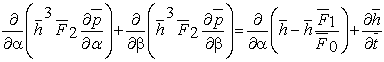(1)

where the non-dimensional functions of viscosity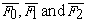are defined, by,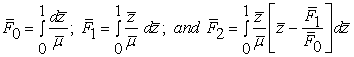(2)

The non-dimensional functions of viscosity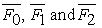report for the effect of variation in fluid viscosity across the film thickness.

Non dimensional minimum film thickness is given by,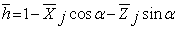(3)

The above equation (1) was solved to satisfy the following boundary and complementary conditions:

i. On the bearing side boundaries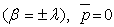(4)

ii. On the supply groove boundaries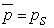(5)

iii. In the positive pressure region, Positive pressures will be generated only when the film thickness is thin,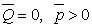(6)

iv. In the cavitated region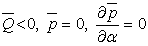(7)

Solution of Eq. (1) gives pressure at each node with above boundary and complementary conditions.

2.1.1. Viscosity-Temperature Equation for Predicting Temperature Distribution in Bearings

The viscosity of fluid film was extremely sensitive to the operating temperature. With increasing temperature the viscosity of fluid decreases. In some cases the viscosity of fluid can fall by about 80% with a temperature increase of 25°C. From the engineering point of view it is important to know the value of viscosity at the operating temperature so it determines the fluid film thickness which separate bush and journal surfaces. The fluid viscosity at a specific temperature can be either calculated from the viscosity-temperature equation or obtained from the viscosity-temperature ASTM chart.

2.1.1.1. Modified Viscosity-Temperature Equation

There were several viscosity-temperature equations available; some of them were purely empirical whereas others were derived from theoretical models. The Vogel equation was the most accurate. In order to keep a machine workable for long periods, friction and wear of its parts must be maintained low. For effective lubrication, fluid must be viscous enough to maintain a fluid film under operating conditions. Viscosity is the most important property of the fluid, which is utilized in hydrodynamic lubrication. The coefficients of viscosity of fluid and density changes with temperature. If a large amount of heat is generated in the fluid film, the thickness of fluid film changes with respect to temperature and viscosity. It is also depend on load. The viscosity of fluid decreases with increasing temperature. Hence, the change in viscosity cannot be ignored. Due to viscous shearing of fluid layer, heat is generated; as significance, high temperatures may be predictable.

Under this condition the fluid can experience a variation in temperature, so that it is necessary to predict the bearing temperature and pressure. Therefore, further investigations on analysis of the thermal effects in journal bearings are needed, so the viscosity-temperature relation given by Ferron J. et al. (3) has been modified. The viscosity μ is a function of temperature and it was assumed to be dependent on temperature. The viscosity of the lubricant was assumed to be variable across the film and around the circumference. The temperature is function of viscosity and it was expressed by Ferron J. et al . The variation of viscosity with the temperature in the non-dimensional two degree equation was described by Ferron J. et al.  this equation was expressed as,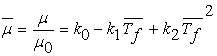(8)

Two degree viscosity-temperature equation was modified and developed into three degree polynomial viscosity-temperature equation. This modified viscosity-temperature equation as illustrated below: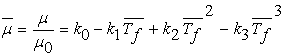(9)

In equation (8) Ferron J. et al.  used the viscosity coefficients as, k0 = 3.287, k1 = 3.064, k2 = 0.777 while the authors considered the following modified viscosity coefficients, k0 = 3.1286, k1 = 2.4817, k2 = 1.1605 and k3 = 0.3266. The polynomial equation was found to acquire improved results.

Results obtained from viscosity-temperature equation developed by authors’ model gives good results when compared with experimental results of Ferron J. et al. . This temperature distribution in an aligned plain journal bearing shows very slight variation between temperature and pressure in journal bearing obtained by authors and temperature obtained by Ferron J. et al. . At different loads the computed maximum bush temperature and pressure are nearly equal for 1500, 2000, 3000 and 4000 rpm. The authors have found during their investigation that the developed viscosity-temperature equation gives very close values of the maximum bush temperature when compared with the experimental results of Ferron J. et al.  at all above mentioned speeds.

2.2. Three Dimensional Energy Equation for Temperature Distribution in Journal Bearing

Three dimensional energy equation is used to determine the temperature distribution in journal bearing. The solution of energy equation needs the pressure field established from solution of Reynolds equation. It is very important to carry out a three-dimensional analysis to accurately predict the temperature distribution in bearings. Accurate prediction of various bearing characteristics, like temperature distribution, is very important in the design of a bearing.

The heat flows inside the solid parts, such as the bush housing of bearing and the shaft, and finally dissipates in the air. The total amount of heat that flows out by convection and conduction is equal to the total amount of heat generated. Temperature distribution in fluid-film is given by three-dimensional energy equation. Fluid temperature has been obtained by solving the following three-dimensional energy equation. This three dimensional energy equation has been modified using thin-film approximation by changing the shape of the fluid film into a rectangular field,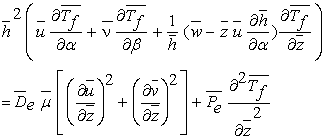(10)

Peclect number (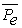) and Dissipation number (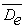) are in non dimensional form, as follows: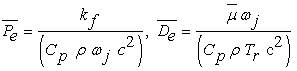(11)

Values of the non-dimensional velocity components in circumferential and axial direction are as follow: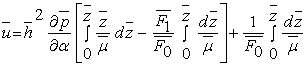(12)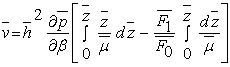(13)

The continuity equation is partially differentiated with respect to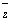to determine the non-dimensional radial component of velocity (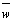) as: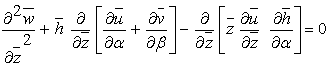(14)

Integrating the above equation with finite difference method considering the following boundary conditions: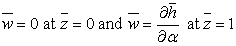(15)

The three dimensional energy equations have been solved with the following boundary conditions:

i. On the fluid–journal interface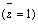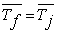(16)

ii. On the fluid–bush interface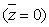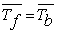(17)
2.3. Heat Conduction Equation for Bush-Housing

Heat conduction analysis was performed to determine the bush temperatures. The Fourier heat conduction equation in the form of non-dimensional cylindrical coordinate form has been solved for the temperature distribution in the bush and is given below: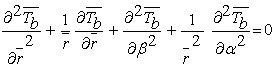(18)

Using the following boundary conditions, heat conduction equation was solved.

i. On the interface of fluid–bush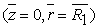:

Continuity of heat flux gives,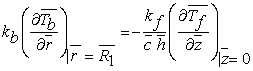(19)

ii. On the outer part of the bush housing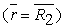:

The free convection and radiation hypothesis gives,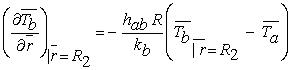(20)

iii. On the lateral faces of the bearing: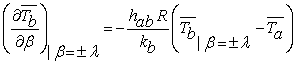(21)

iv. At the outlet edge of bearing pad, free convection of heat flow from bush to fluid in the supply groove gives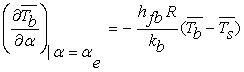(22)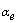= Circumferential coordinate of the outlet edge of bearing.

v. At the inlet edge of the bearing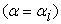and at the fluid supply point on the outer surface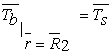(23)

In addition, a free convection of heat between fluid and housing has been assumed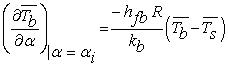(24)

Where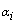= circumferential coordinate of the inlet edge of bearing.

2.4. Heat Conduction Equation for Journal

For finding the temperature distribution in journal, the following assumptions were made,

i. Conduction of heat in the axial direction.

ii. Journal temperature does not vary in radial or circumferential direction at any section.

iii. Heat flows out of the journal from its axial ends. Hence the following steady state unidirectional heat conduction equation was used for a journal: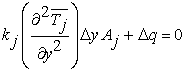(25)

Where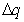= the heat input to the element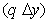;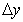= the length of element.

The above equation reduces to the following non-dimensional form: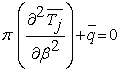(26)

whereis the non-dimensional heat input to journal per unit length.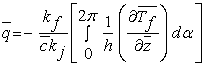(27)

The above equations have been solved with the following boundary condition:

At the axial ends, i.e.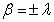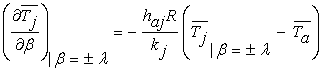(28)
2.5. Thermal Mixing of hot Recirculating Fluid with Incoming cold Fluid in a Supply Groove

It was not possible for the experimenters to maintain the inlet fluid temperature at a constant value. Because of low supply pressures and high fluid viscosities, the inlet fluid temperature will decrease. Incoming fluid temperature is less as compared to recirculating temperature of fluid. Thermal mixing analysis of hot recirculating and incoming cold fluid from supply groove was used to calculate the fluid temperature at the inlet of the groove. Energy balance equation is used to estimate the mean temperature of the fluid in a groove.

In this work, the overall energy balance equation is expressed in terms of mean temperature, Tm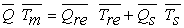(29)

where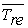recirculating hot fluid, for the unit length of bearing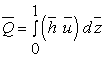(30)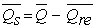(31)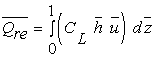(32)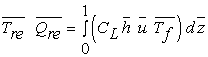(33)

Mean temperature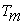related to the assumed temperature distribution,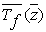across the fluid film at the inlet of the bearing pad as below: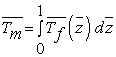(34)

#### Table 1. Operating ConditionsDownload asPowerPoint Slide

Veiw figureView current table in a new window

### 3. Solution Procedure

The solution of Reynolds equation requires the viscosity of fluid, which is obtained from energy equation. Reynolds equation solved by finite element method for obtaining pressure distribution in the fluid-film by iterative technique. The negative pressure nodes were set to zero. Altitude angle was modified till convergence was achieved. Pressure and temperature fields for the initial eccentricity ratio have been recognized. Velocity components of the fluid film were calculated in circumferential, axial and radial directions. Coefficient of contraction of fluid-film was determined. In the positive pressure region Coefficient of contraction was assumed as unity.

Finite difference method was used to solve the three dimensional energy equations for temperature distribution in the fluid film and bush housing. One dimensional heat conduction equation was used for temperature distribution in journal. The journal temperature was revised after obtaining the converged temperature for fluid and bush. The energy and Fourier conduction equations were simultaneously solved with revised journal temperature. All the above steps were repeated until the convergence was achieved.

### 4. Results and Discussion

Numerical calculations were performed by writing a computer program in C. The governing equations as Reynolds equation and three dimensional energy equations were discretized for numerical solution. The global iterative scheme was used. Four nodes element was used for this discretization. A mesh discretization for fluid film and bush with 68 nodes in the circumferential direction, 10 nodes in the axial direction and 10 nodes across the film thickness and 10 nodes across the radius of bush thickness were used for Finite Element Method and Finite Difference Method. Table 1 depicts the input parameters used for thermohydrodynamic analysis of plain journal bearing. The present assumes aligned plain journal bearing. The values of coefficient for the parabolic distribution were evaluated by considering temperature of fluid equal to temperature of bush at the fluid–bush interface. Journal temperature is equal to the fluid–journal interface. The condition of mixing the recirculating fluid with the supply fluid was also included.

Figure 1 depicts the distribution of the maximum bush temperature obtained with different eccentricity ratio for different speeds.

Figure 1. Maximum bush temperature versus eccentricity ratio for different speeds

The experimental maximum bush temperature is nearly equal to theoretical values as per modified viscosity-temperature equation. The bush temperature increases with load.

Figure 2 and Figure 3 predicts the circumferential temperature distribution in the mid-plane of fluid-bush interface. The authors compared their theoretical results from the developed equation of viscosity-temperature relation, with the experimental data of published results. Theoretical predictions and experimental results of Ferron J. et al.  exhibit a similar pattern, the predicted maximum temperature value and their locations are reasonably very close to the measured values of Ferron J.et al.  Pressure variation in mid plane of plain journal bearing for various speeds and loading conditions were shown in the Figure 4 and Figure 5.

Figure 6. Variation in mean journal temperature at different loads and 2000 rpm, 4000 rpm speeds.

Pressure distribution was negative in diverging region where as in converging region there was positive pressure. In the authors developed model pressure distribution was very close to the experimental values given by Ferron J. et al. . Theoretical results predicted by modified viscosity temperature equation and experimental results concerning pressure in good agreement. The mean journal temperature has been computed along axial direction. Figure 6 depicts load versus mean journal temperature at 2000 rpm and 4000 rpm. Figure 6 indicates that the present theoretical results by using modified viscosity-temperature model are very close to experimental results with the published results of Ferron J. et al. . The modified viscosity-temperature equation gives values of maximum fluid-bush interface temperature very closer to the experimental values of Ferron J et al. .The radial temperature was negligible. In the present work journal temperature along axial direction of the journal varies by about one degree for 2000 rpm and very close to the 4000 rpm at two different loads 4000 N and 6000 N respectively

### 5. Conclusions

A theoretical study of modified viscosity and temperature equation on an aligned plain journal bearing has been made. On the basis of results and discussions presented in the earlier sections, the following major conclusions are drawn:

•  The modified viscosity-temperature equation for this work is more appropriate.

•  The modified viscosity-temperature model which was used in this study will predict the temperature and pressure in the plain journal bearing.

•  The values of maximum bush temperature and maximum fluid pressure in the mid-plane of the bearing, occur near the minimum film thickness.

•  The temperature and pressure of fluid-film increases with increase in load and speed of journal.

•  When the bearing is highly loaded, the thermal effects should be considered. The viscous heating in high speed bearings reduces the load carrying capacity.

•  Due to increase in temperature of the fluid-film, the load carrying capacity decrease significantly. Hence, proper arrangements have to be made to cool the recirculating fluid to maintain the load carrying capacity of journal bearing.

•  The effect of mixing of recirculating and supply temperatures of fluid in the groove is quite important.

•  It has been shown that effect of variation of viscosity and temperature in journal bearing cannot be neglected.

•  The modified equation by authors give very close values of the maximum fluid-film and bush temperature when compared with the experimental values of Ferron J. et al.  at different speeds and loads respectively.

### References

  Allan, T., “The application of finite element analysis to hydrodynamic and externally pressurised pocket bearings,” Wear, 19, 169-206, 1972.In article CrossRef  Dowson, D. and Ashton, J. N., “Optimum computerized design of Hydrodynamic Journal Bearings,” International Journal of Mech. Sciences, 18, 215-222, April, 1976.In article CrossRef  Ferron, J., Frene, J. and Boncompain, R. A., “Study of thermohydrodynamic performance of a plain journal bearing Comparison between theory and experiments,” ASME Journal of Lubrication Technology, 105, 422-428, July, 1983.In article CrossRef  Heshmat, H. and Pinkus, O., “Mixing inlet temperature in hydrodynamic bearings,” ASME Journal of Tribology, 108, 231-248, 1986.In article CrossRef  Chandrawat, H. N. and Sinhasan, R. A., “Comparison between two numerical techniques for hydrodynamic journal bearing problems,” Wear, 119, 77-87, March, 1987.In article CrossRef  Khonsari, M. M. and Beaman, J. J., “Thermohydrodynamic analysis of laminar incompressible journal bearings,” ASLE Transactions, 29 (2), 141-150, 1987.In article CrossRef  Banwait, S. S. and Chandrawat, H. N., “Study of thermal boundary conditi0.ons for a plain journal bearing,” Tribology International, 31, 289-296, May, 1998.In article CrossRef  Costa, L., Fillon, M., Miranda, A. S. and Claro, J. C. P., “An Experimental Investigation of the Effect of Groove Location And Supply Pressure on the THD Performance of a Steadily Loaded Journal Bearing,” ASME Journal of Tribology, 122, 227-232, 2000.In article CrossRef  Tanaka, M., “Journal bearing performance under starved lubrication,” Tribology International, 33, 259-264, 2000.In article CrossRef  Sang, Myung Chun and Dae-Hong Ha, “Study on mixing flow effects in a high-speed journal bearing,” Tribology International, 34, 397-405, March, 2001.In article CrossRef  Tanaka, M. and Hatakenaka, K., “Turbulent thermohydrodynamic lubrication models compared with measurements,” Proceedings of the Institution of Mechanical Engineers, Part J: Journal of Engineering Tribology, 218, 391-399, June, 2004.In article CrossRef  Kosasih, P. B. and Tieu, A. K., “An investigation into the thermal mixing in journal bearings,” Proceedings of the Institution of Mechanical Engineers, Part J: Journal of Engineering Tribology, 218, 379-389, June, 2004.In article CrossRef  Singh, D. S. and Majumdar, B. C., “Computer-aided design of hydrodynamic journal bearings considering thermal effects,” Proceedings of the Institution of Mechanical Engineers, Part J: Journal of Engineering Tribology, 219, 133, January, 2005.In article CrossRef  Fillon, M. and Bouyer, J., “Thermohydrodynamic analysis of a worn plain journal bearing;” Tribology International, 37, 129-136, 2004.In article CrossRef  Jeddi, L., Khlifi, M. El. and Bonneau, D., “Thermohydrodynamic analysis for a hydrodynamic journal bearing groove,” I Mech. E, Part J: J. Engineering Tribology Proceeding, 219, 263-274, May, 2005.In article  Banwait, S. S., “A Comparative Performance Analysis of Non-circular Two-lobe and Three-lobe Journal Bearings,” IE (I) I Journal-MC, 86, 202-210, March, 2006.In article  Singh, U., Roy, L. and Sahu, M., “Steady-state thermo-hydrodynamic analysis of cylindrical fluid film journal bearing with an axial groove,” Tribology International, 41, 1135-1144, 2008.In article CrossRef  Roy, L., “Thermo-hydrodynamic performance of grooved oil journal bearing,” Tribology International, 42, 1187-1198, 2009.In article CrossRef  Maneshian, B. and Gandjalikhan, Nassab S. A., “Thermohydrodynamic Characteristics of Journal Bearings Running Under Turbulent Condition.” IJE Transactions A: Basics, 22 (2), 181-194, June, 2009.In article  Maneshian, B. and Gandjalikhan, Nassab S. A., “Thermohydrodynamic analysis of turbulent flow in journal bearings running under different steady conditions,” Engineering Tribology proc. Part J, I Mech E, 223, 1115-1127. February 2009.In article  Panday, K. M., Choudhury, P. L., and Kuma, N. P., “Numerical Unsteady Analysis of Thin Film Lubricated Journal Bearing, ” IACSIT International Journal of Engineering and Technology, Vol. 4, No. 2, 185-191, April, 2012.In article CrossRef  Mukesh, Sahu, Ashish, Kumar Giri and Ashish Das, “Thermohydrodynamic Analysis of a Journal Bearing Using CFD as a Tool.” International Journal of Scientific and Research Publications; 2 (9), 1-7, 2012.In article  Sujith, Prasad E., Nagaraju, T. and Prem, sagar J., “Thermohydrodynamic performance of a journal bearing with 3d-surface roughness and fluid inertia effects,” International Journal of Applied Research in Mechanical Engineering (IJARME) ISSN; 2 (1), 2231-5950, 2012.In article  Abdessamed, Nessil, Salah, Larbi, Hacene Belhaneche and Maamar, Malki, “Journal Bearings Lubrication Aspect Analysis Using Non-Newtonian Fluids,” Advances In Tribology, 1-10, 2013.In article  Brito, F.P., Miranda, A.S., Claro, J. C. P, Teixeira, J.C., Costa, L. and Fillon, M., “The role of lubricant feeding conditions on the performance improvement and friction reduction of journal bearings,” Tribology International, 72, 65-82. 2014.In article CrossRef  Kadam, Kanifnath, Banwait, S. S. and Laroiya, S. C., “Thermohydrodynamic Analysis of Plain Journal Bearing with Modified Viscosity-Temperature Equation,” International Journal of Mechanical Engineering and Technology (IJMET), Volume 5, Issue 11, 31-46, November, 2014.In article

### Nomenclature